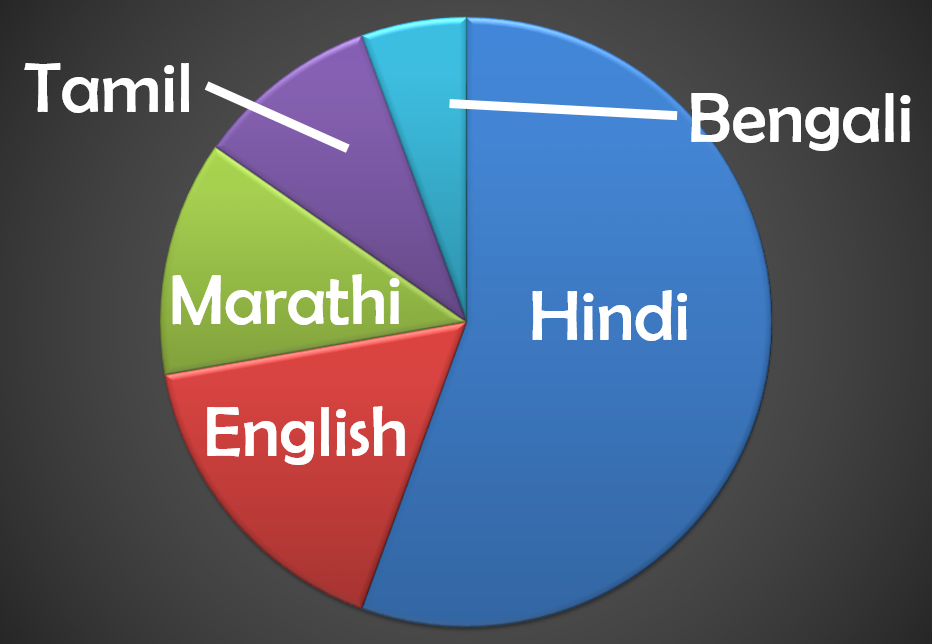# vs.eyeandcontacts.com

## Chapter 5 Data Handling Exercise 5.2

Question 1: A survey was made to find the type of music that a certain group of young people liked in a city.
Adjoining pie chart shows the findings of this survey. From this pie chart, answer the following:
(i) If 20 people liked classical music, how many young people were surveyed?
(ii) Which type of music is liked by the maximum number of people?
(iii) If a cassette company were to make 1000 CD’s, how many of each type would they make?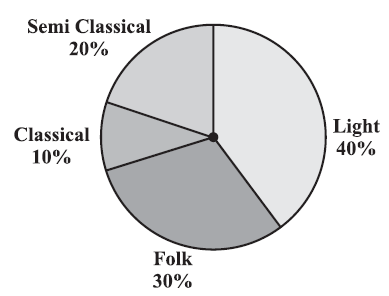i)
Number of people who liked classical music = 20
Percentage of people like classic music = 10%
Number of people surveyed = ?
10% of x = 20
10x/100 = 20
x = 200
Therefore, 200 people were surveyed.

ii) Light music is liked by the maximum number of people.

iii) CD’s = 1000

Therefore, 200 CD’s were made for Semi classical, 100 CD’s were made for Classical, 300 CD’s were made for Folk and 400 CD’s were made for Light Music.

Question 2: A group of 360 people were asked to vote for their favorite season from the three seasons rainy, winter and summer.
(i) Which season got the most votes?
(ii)Find the central angle of each sector.
(iii) Draw a pie chart to show this information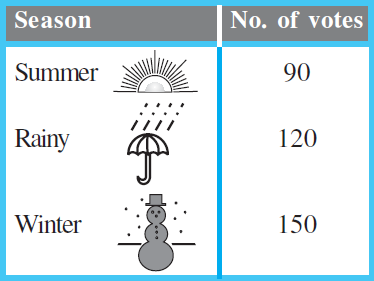i) Winter season got the most votes.

ii)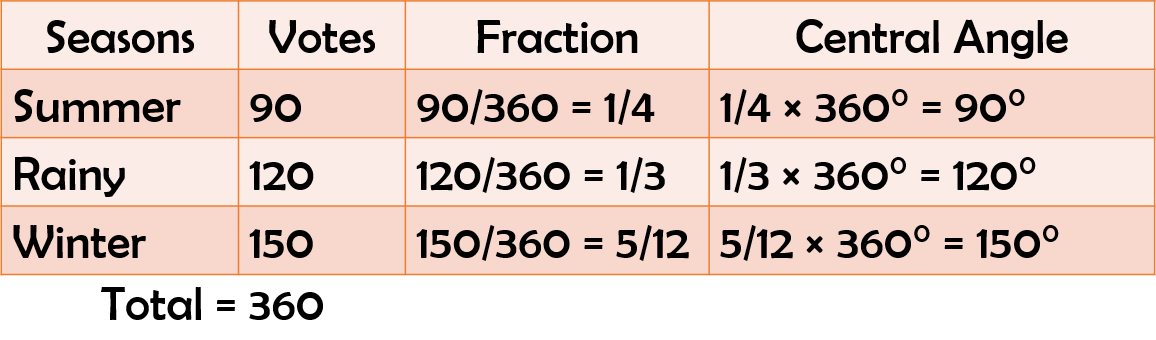Therefore, the central angle of summer is 90°, the central angle of rainy is 120° and the central angle of winter is 150°.

iii)Question 3: Draw a pie chart showing the following information. The table shows the colours preferred by a group of people.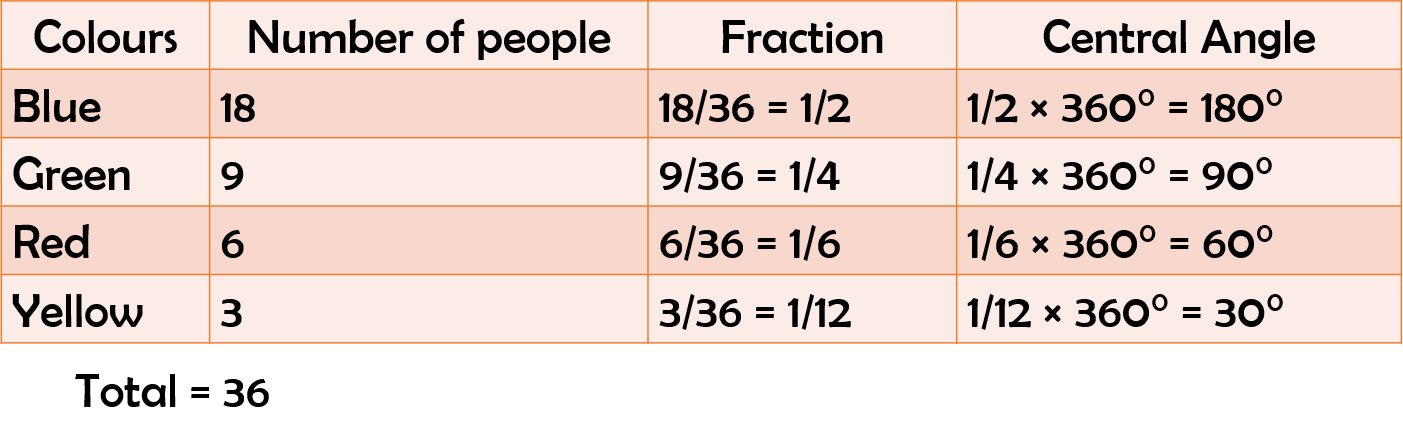Question 4: The adjoining pie chart gives the marks scored in an examination by a student in Hindi, English, Mathematics, Social Science and Science. If the total marks obtained by the students were 540, answer the following questions.
(i) In which subject did the student score 105 marks?
(Hint: for 540 marks, the central angle = 360°. So, for 105 marks, what is the central angle?)
(ii) How many more marks were obtained by the student in Mathematics than in Hindi?
(iii) Examine whether the sum of the marks obtained in Social Science and Mathematics is more than that in Science and Hindi. (Hint: Just study the central angles).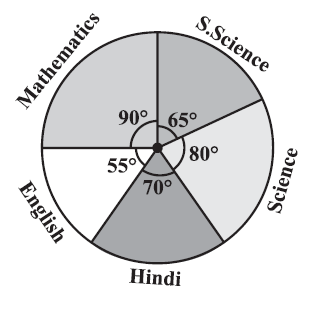i)
Total marks = 540
105/540 × 360° = 70°
Hindi = 105 marks
Therefore, the student scored 105 marks in Hindi.

ii)
= 90/360 × 540 = 135 marks (Maths)
= 135 - 105 = 30 marks
Therefore, the student scored 30 marks more in Maths than Hindi.

iii)
= 90/360 × 540 = 135 marks (Maths)
= 65/360 × 540 = 97.5 marks (Social)
= 80/360 × 540 = 120 marks (Science)
= 70/360 × 540 = 105 marks (Hindi)
Compare
135 + 97.5 = 232.5
120 + 105 = 225
232.5 > 225

Therefore, Sum of marks obtained in Maths & Social is more than Science & Hindi.

Question 5: The number of students in a hostel, speaking different languages is given below. Display the data in a pie chart.